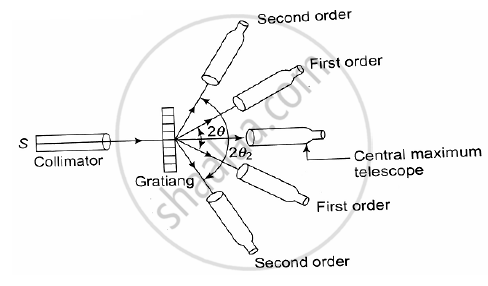# Explain the Experimental Method to Determine the Wavelength of Spectral Line Using Diffraction Grating. - Applied Physics 2

Explain the experimental method to determine the wavelength of spectral line using diffraction grating.
What is the highest order spectrum which can be seen with monochromatic light of wavelength 6000 Ao by means of a diffraction grating with 5000 lines/cm?

#### Solution1.The grating spectrum of the given source of monochromatic light is obtained by using a spectrometer.

2.The arrangement is as shown in Figure shown below.

3.The spectrometer is first adjusted for parallel rays.

4.The grating is then placed on the prism table and adjusted for normal incidence.

5.In the same direction as that of the incident light, the direct image of the slit or the zero-order spectrum can be seen in the telescope.

6.On either side of this direct image a symmetrical diffraction pattern consisting of different orders can be seen.

7.The angle of diffraction θ for a particular order m of the spectrum is measured.

8.Thus using the equation (a + b) sin θ = mλ the unknown wavelength λ can be calculated by putting the values of the grating element (a + b), the order m and the angle of diffraction θ.

9. The diffraction grating is often used in the laboratories for the determination of wavelength of light.

Solution of problem :

N = 5000 ("lines")/(cm)

a + b = 1/5000 cm

"Using equation (a + b)sin θ = mλ"

For m to be maximum sin θ should be equal to 1

n = (a+b)/λ

= 1/(5000 xx 6000 xx 10^(−8) )

= 3.3

Maximum order of spectrum = 3

Concept: Diffraction Grating
Is there an error in this question or solution?